Main Content

## Inspect Variable-Size Signals on Simulink Models

### Variable-Size Signal Generation and Operations

This example model shows how to create a variable-size signal from multiple fixed-size signals and from a single data signal. It also shows some of the operations you can apply to variable-size signals.

For a complete list of blocks that support variable-size signals, see Simulink Block Support for Variable-Size Signals.

1. In the MATLAB® Command Window, type

`sldemo_varsize_basic`

2. In the Simulink® Editor, on the Debug tab, select Information Overlays > Signal Dimensions. Run a simulation or press Ctrl-D.

The Simulink Editor displays the signal dimensions and line styles. See Signal Basics for an interpretation of signal line styles.

3. So that you can see the names of the blocks in the model, on the Format tab, clear Auto > Hide Automatic Block Names.#### Create a Variable-Size Signal from Fixed-Size Signals

One way to create a variable-size signal is to use the Switch block. The input signals to the Switch block can differ in their number of dimensions and in their size.Output from the Switch block is a 2-D variable-size signal with a maximum size of `3x2`. When you select the Allow different data input sizes parameter on the Switch block, Simulink does not expand the scalar value from the Constant1 block.

#### Save Variable-Size Signal Data

You could add a To Workspace block to the output from the Switch block. Since the model already has a To Workspace block, the second To Workspace block would save data to a signal array named `simout2 `The `values` field logs the actual signal values. If logged signal data is smaller than the maximum size, values are padded with NaNs or appropriate values. To obtain these signal values, type:

```simout2.signals.values ans(:,:,1) = 1 -1 -2 2 -3 3 ans(:,:,2) = 1 -1 -2 2 -3 3 ans(:,:,3) = 0 NaN NaN NaN NaN NaN```

The `valueDimensions` field logs the dimensions of a variable-size signal. To obtain the dimensions, type:

```simout2.signals.valueDimensions ```

The signal dimensions for the first three time steps are shown.

```ans = 3 2 3 2 1 1 ```

#### Create a Variable-Size Signal from a Single Data Signal

The data signal (Constant5) is a `3x4` matrix. The Pulse Generator represents a control signal that selects a starting and ending index value ( ```[1 2]``` or `[1 3]`). The Selector block then uses the index values to select different parts of the data signal at each time step and output a variable-size signal.#### View Changes in Signal Size

The output from the Selector block is either a `2x2` or `3x3` matrix. Because the maximum dimension for a variable-size signal is the `3x4` matrix from the data signal, the logged output signals are padded with NaNs.

Use the Probe or Width blocks to inspect the current dimensions and width of a variable-size signal. In addition, you can display variable-size signals on Scope blocks and save variable-size signals to the workspace using the To Workspace block.

#### Process Variable-Size Signals

The remainder of the model shows various operations that are possible with variable-size signals. Operations include using the Gain, Sum, Math Function, and Matrix Concatenate blocks. You can connect variable-size signals with the From, Goto, Bus Assignment, Bus Creator, and Bus Selector blocks.

### Variable-Size Signal Length Adaptation

This example model corresponds to a hypothetical system where the model adapts the length of a signal over time. Length adaptation is based on the value of a control signal. When the control signal falls within one of three predefined ranges, the fixed-size raw data signal changes to a variable-size data signal.

The variable-size signal connects to a processing block, where blocks that support variable-size signals operate on it. A MATLAB Function block with both input and output signals of variable size allow more flexibility than other blocks supporting variable-size signals. See Simulink Block Support for Variable-Size Signals.

To open the example model, in the MATLAB Command Window, type:

`sldemo_varsize_dataLengthAdapt`So that you can see the names of the blocks, in the model, on the Format tab, clear Auto > Hide Automatic Block Names.

#### Creating a Variable-Size Signal by Adapting the Length of a Data Signal

This model generates a data signal and converts the signal to a variable-size signal. The size of the signal depends on the value of a control signal. The raw data signal is a column vector with values from `1` to `9`.

```[1:9].' ans = 1 2 3 4 5 6 7 8 9```
The Size Selection subsystem determines the quality of the data signal and outputs a quality value ( 1, 2, or 3). This value helps to select the length of the data signal in the Length Adaptation subsystem.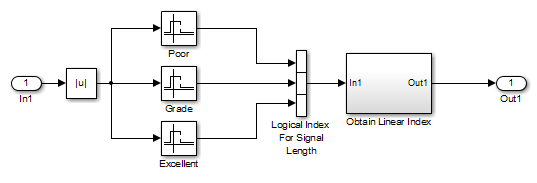In the Length Adaptation subsystem, the Signal Size subsystem generates an index based on the quality value from the Size Selection subsystem (In2). The Data Selector block uses the starting and ending indices to adapt the length of the data signal (In1) and output a variable-size signal.#### Processing a Variable-Size Signal

The center section of the model processes the variable-size signal. The MATLAB Function block adds zeros between the data values in a way that is similar to upsampling a signal. The dimension of the signal changes from `9` to `18`. The Math Function blocks show various manipulations you can do with variable-size signals.#### Visualizing a Variable-Size Signal

The right section of the model determines the signal width (size) and uses a scope to visualize the width and the processed data signal.### Mode-Dependent Variable-Size Signals

This example model represents a system that has three operation modes. For each mode, the data signal to process has a different size.

The Process subsystem in this model receives a variable-size signal where the size of the signal depends on the operation mode of the system. For each mode change, the Stateflow® chart, Mode Control Logic, detects when the data signal size changes. It then generates a function call to reset the blocks in the Process subsystem.

To open the model, In the MATLAB Command Window, type:

`sldemo_varsize_multimode`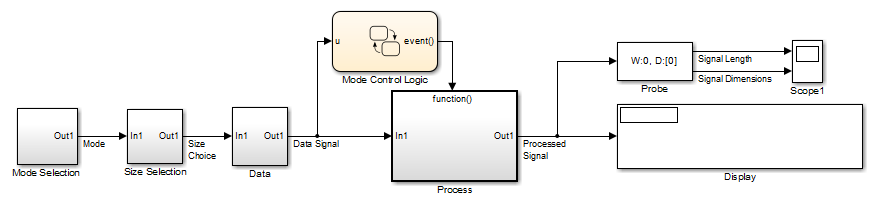So that you can see the names of the blocks, in the model, on the Format tab, clear Auto > Hide Automatic Block Names.

#### Creating a Variable-Size Signal Based on Mode

The Mode Selection subsystem determines the mode for processing a data signal and outputs a mode value ( 1, 2, or 3). This value helps to select the length of the data signal using the Size Selection and Data subsystems.The Size Selection subsystem creates an index value from the mode value. In this example, the index values are `[1 3]`, ```[1 2]```, and `[1 1]`.The Data subsystem takes a data signal (Constant block) and selects part of the data signal dependent on the mode. The output is a variable-size signal with a matrix size of `3x3`, `2x2`, and `1x1`.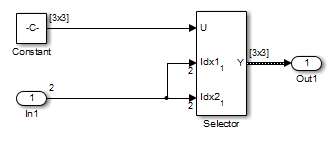The dimensions of the raw data signal (Constant block) is a `3x3`. After connecting a To Workspace block to a signal line, you can view the signal in the MATLAB Command Window by typing:

```simout.signals.values ans(:,:,1) = 1 4 7 2 5 8 3 6 9 ```

The variable-size signal generated from the Data subsystem is also a `3x3` matrix. For shorter signals, the matrix is padded with NaNs.

```simout.signals.values ans(:,:,1) = 1 NaN NaN NaN NaN NaN NaN NaN NaN ans(:,:,2) = 1 4 NaN 2 5 NaN NaN NaN NaN ans(:,:,3) = 1 4 7 2 5 8 3 6 9```

#### Processing a Variable-Size Signal with a Conditionally Executed Subsystem

Because the Process subsystem contains a Delay block, the subsystem resets and repropagates the signal at each time step. This model uses a Stateflow chart to detect a signal size change and reset the Process subsystem.

In the function block dialog, and from the Propagate sizes of variable-size signals list, choose `Only when enabling`. When the model enables this subsystem, selecting this option directs the Simulink software to propagate sizes for variable-size signals inside the conditionally executed subsystem. Signal sizes can change only when they transition from disabled to enabled. For an explanation of handling signal-size changes with blocks containing states, see How Variable-Size Signals Propagate.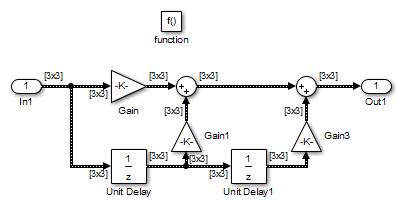The Stateflow chart determines if there is a change in the size of the signal. The function `size_detect` calculates the width of the variable-size signal at each time step, and compares the current width to the previous width. If there is a change in signal size, the chart outputs a function-call output event that resets and repropagates the signal sizes within the Process subsystem.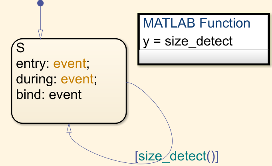#### Visualizing Data

Use the Probe block to visualize signal size and signal dimension.Since the signals are ```n x n``` matrices, the signal dimension lines overlap in the Scope display.You can use a Display block and the Simulink Debugger to visualize signal values at each time step.### S-Functions Using Variable-Size Signals

#### Level-2 MATLAB S-Function with Variable-Size Signals

Both Level-2 MATLAB S-Functions and C S-Functions support variable-size signals when you set the DimensionMode for the output port to `Variable`. You also need to consider the current dimension of the input and output signals in the input and output update methods.

To open this example model, in the MATLAB Command Window, type:

`msfcndemo_varsize`The Enabled subsystem includes a Level-2 MATLAB S-Function which shows how to implement a block that holds its states until reset. Because this block contains states and delays the input signal, the input size can change only when a reset occurs.

The Expand block is a Level-2 MATLAB S-Function that takes a scalar input and outputs a vector of length indicated by its input value. The output is by `1:n` where `n` is the input value.

#### C S-Function with Variable-Size Signals

To open this example model, in the MATLAB Command Window, type:

`sfcndemo_varsize`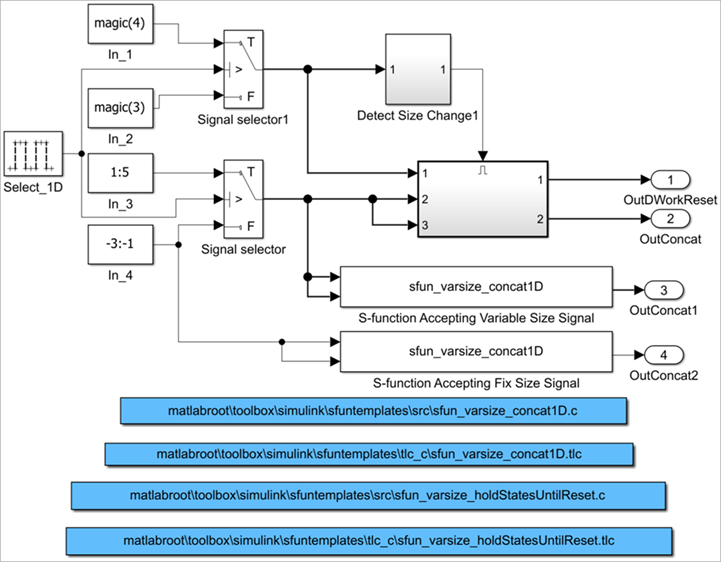The enabled subsystems have two S-Functions:

• sfun_varsize_holdStatesUntilReset is a C S-Function that has states and requires its DWorks vector to reset whenever the sizes of the input signal changes.

• sfun_varsize_concat1D is a C S-function that implements the concatenation of two unoriented vectors. You can use this function within an enabled subsystem by itself.

## Support

#### Model-Based Design for Embedded Control Systems

Download white paper